# How to use H-bar(ℏ) symbol in LaTeX?

This ℏ letter from the Latin alphabet is used in physics as a symbol of the Planck constant, and it is also used as a symbol for Hedera Hashgraph’s native cryptocurrency. How to use this symbol in Latex will be discussed in this tutorial.

SymbolH-bar
Type of symbolMathematics
Package (requirement)No
ArgumentNo
Latex command\hbar
Example\hbar → ℏ

LaTeX provides a command to print the h-bar symbol in a LaTeX document, which is \hbar. This command lets you easily print the h-bar symbol to the document and is a default command in LaTeX. Since this command works in math mode, you must always enclose the command in single-dollar($) or $...$. Below are some examples of the use of \hbar command. \documentclass{article} \begin{document} $J_z = m \hbar$ $\hbar = \frac{h}{2\pi}$ $p^N = \hbar \left(\frac{w}{c}, \vec{K} \right)$ \end{document} Output :## H-bar symbol using other packages You can also use other packages to print h-bar symbols in latex documents. Some packages use a similar command as the default command in \hbar, and some packages use a slightly different command to print the h-bar symbol to the document. But you can see some differences in the h-bar symbols of different packages in the output. The following table shows how the h-bar symbol will appear in various packages, commands, and output. PackageCommand and Output amsmath\hbar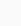fdsymbol\hbar →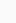boisik\hbar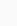boisik\hslash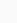phonetic\planckwsuipa\crossh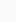tipa\textcrh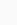To use this symbol in text mode, you can use the \planck command from the phonetic package or the \textcrh command from the tipa package. The following example shows the use of these two packages. \documentclass{article} \usepackage{phonetic,tipa} \begin{document} E=\planck$\omega  $\\[4pt] \textcrh=$1.054571817\times 10^{-34} \$ J-s
\end{document}

Output :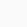### 1 thought on “How to use H-bar(ℏ) symbol in LaTeX?”

1.Gal Jerman

WOOOOOPPPP ,…. top post ,.. gona save it!

Scroll to Top